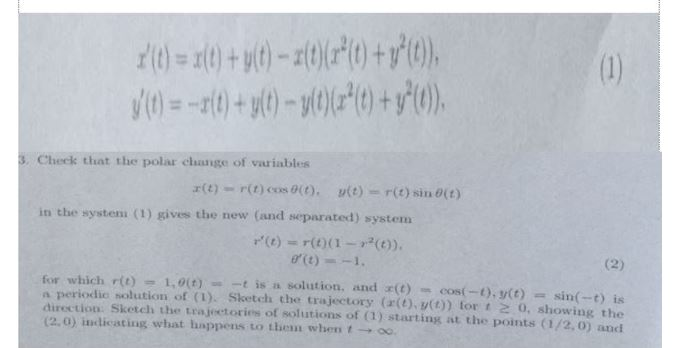# 3. Check that the polar change of variables x(t)-r(t)(os0(t), y(t) = r(t) sin0(t) in the system (1) gives the new (and separated) systenm r(t) = r(t)(1-r2(t)), for which r(t) 1,0(t)--t is n solution, and (t)-cos(-t), y(t) = sin(-t) is a periodic solution of (1). Sketch the trajectory (a(t) v(t)) tor t 2 0, showing the direction: Sketch the trajectories of solutions of (1) starting at the points (1/2, 0) andi (2,0) įlidicating what happens to Lhem when t → oo.

Question

pls explain to me step by step. pls pls dont skip any steps. thankshelp_outlineImage Transcriptionclose3. Check that the polar change of variables x(t)-r(t)(os0(t), y(t) = r(t) sin0(t) in the system (1) gives the new (and separated) systenm r(t) = r(t)(1-r2(t)), for which r(t) 1,0(t)--t is n solution, and (t)-cos(-t), y(t) = sin(-t) is a periodic solution of (1). Sketch the trajectory (a(t) v(t)) tor t 2 0, showing the direction: Sketch the trajectories of solutions of (1) starting at the points (1/2, 0) andi (2,0) įlidicating what happens to Lhem when t → oo. fullscreen

## Expert Answer

### Want to see this answer and more?

Experts are waiting 24/7 to provide step-by-step solutions in as fast as 30 minutes!*

*Response times vary by subject and question complexity. Median response time is 34 minutes and may be longer for new subjects.
Tagged in© 2021 bartleby. All Rights Reserved.